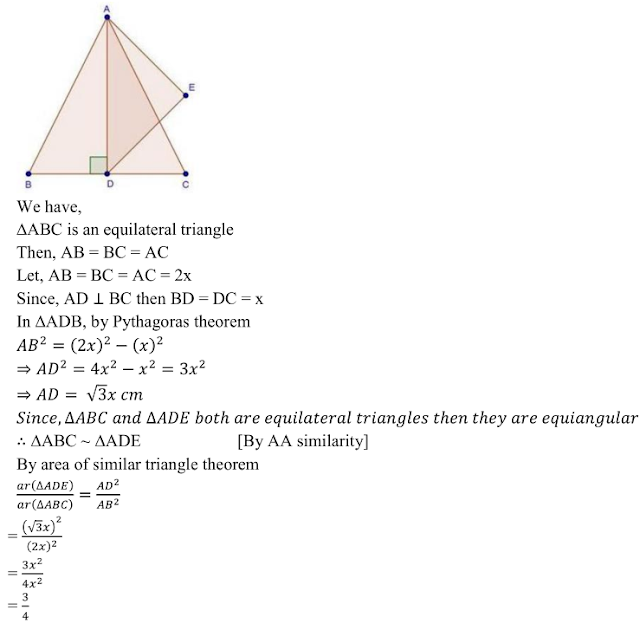## Chapter 4 triangles R.D. Sharma Solutions for Class 10th Math Exercise 4.6

Exercise 4.6

1. Triangles ABC and DEF are similar
(i) If area (△ABC) = 16cm2, area (△DEF) = 25 cm2 and BC = 2.3 cm, find EF.
(ii) If area (△ABC) = 9cm2, area (△DEF) = 64 cm2 and DE = 5.1 cm, find AB.
(iii)If AC = 19cm and DF = 8 cm, find the ratio of the area of two triangles.
(iv)If area (△ABC) = 36cm2, area (△DEF) = 64 cm2 and DE = 6.2 cm, find AB.
(v) If AB = 1.2 cm and DE = 1.4 cm, find the ratio of the areas of △ABC and △DEF.

Solution

(i)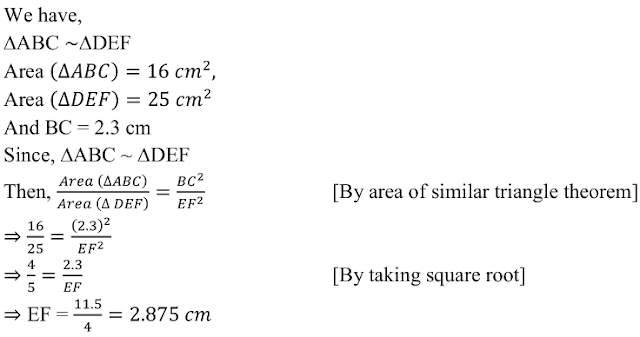(ii)(iii)(iv)(v)2. In fig. below ∆ACB ~ ∆APQ. If BC = 10 cm, PQ = 5 cm, BA = 6.5 cm and AP = 2.8 cm, find CA and AQ. Also, find the area (∆ACB): area (∆APQ).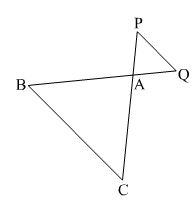Solution3. The areas of two similar triangles are 81 cm2 and 49 cm2 respectively. Find the ratio of their corresponding heights. What is the ratio of their corresponding medians ?

Solution4. The areas of two similar triangles are 169 cm2 and 121 cm2 respectively. If the longest side
of the larger triangle is 26 cm, find the longest side of the smaller triangle.

Solution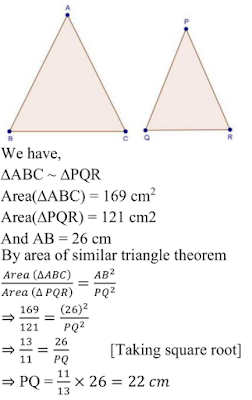5. The areas of two similar triangles are 25 cm2 and 36 cm2 respectively. If the altitude of the first triangle is 2.4 cm, find the corresponding altitude of the other.

Solution6. The corresponding altitudes of two similar triangles are 6 cm and 9 cm respectively. Find the ratio of their areas.

Solution7. ABC is a triangle in which ∠A =90°, AN⊥ BC, BC = 12 cm and AC = 5cm. Find the ratio of the areas of ∆ANC and ∆ABC.

Solution8. In Fig. 4.178, DE || BC
(i) If DE = 4 cm, BC = 6 cm and Area (∆ADE) = 16 cm2 , find the area of ∆ABC.
(ii) If DE = 4cm, BC = 8 cm and Area (∆ADE) = 25 cm2 , find the area of ∆ABC.
(iii)If DE : BC = 3 : 5. Calculate the ratio of the areas of ∆ADE and the trapezium BCED .Solution9.In ∆ABC, D and E are the mid-points of AB and AC respectively. Find the ratio of the areas of ∆ADE and ∆ABC .

Solution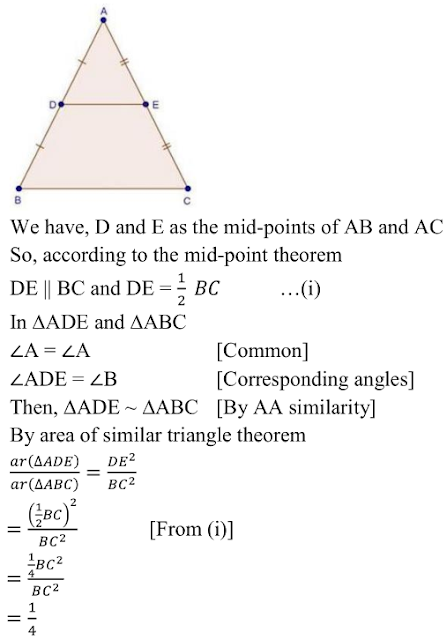10. The areas of two similar triangles are 100 cm2 and 49 cm2 respectively. If the altitude the
bigger triangle is 5 cm, find the corresponding altitude of the other .

Solution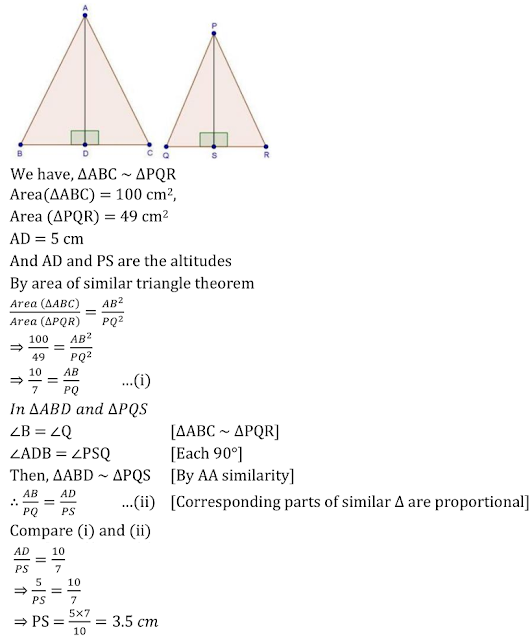11. The areas of two similar triangles are 121 cm2 and 64 cm2 respectively. If the median of the
first triangle is 12.1 cm, find the corresponding median of the other.

Solution12. If ∆ABC ~ ∆DEF such that AB = 5 cm, area (∆ABC) = 20 cm2 and area (∆DEF) = 45 cm2 , determine DE.

Solution13. In ∆ABC, PQ is a line segment intersecting AB at P and AC at Q such that PQ || BC and PQ divides ∆ABC into two parts equal in area. Find BP/AB .

Solution14. The areas of two similar triangles ABC and PQR are in the ratio 9:16. If  BC = 4.5 cm, find the length of QR.

Solution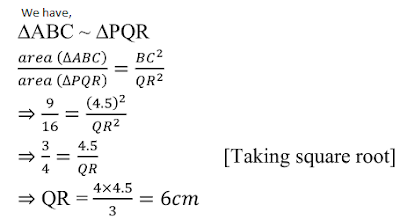15. ABC is a triangle and PQ is a straight line meeting AB in P and AC in Q. If AP = 1 cm, PB = 3 cm, AQ = 1.5 cm, QC = 4.5 m, prove that area of ∆APQ is one- sixteenth of the area of ABC.

Solution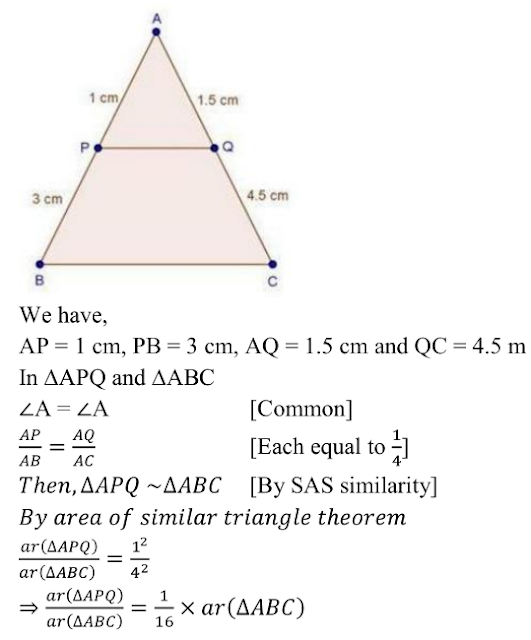16. If D is a point on the side AB of ∆ABC such that AD : DB = 3.2 and E is a Point on BC such that DE || AC. Find the ratio of areas of ∆ABC and ∆BDE.

Solution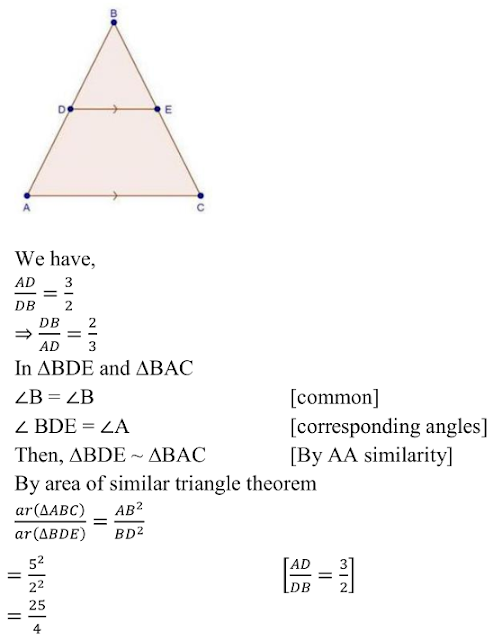17. If ∆ABC and ∆BDE are equilateral triangles, where D is the mid-point of BC, find the ratio of areas of ∆ABC and ∆BDE.

Solution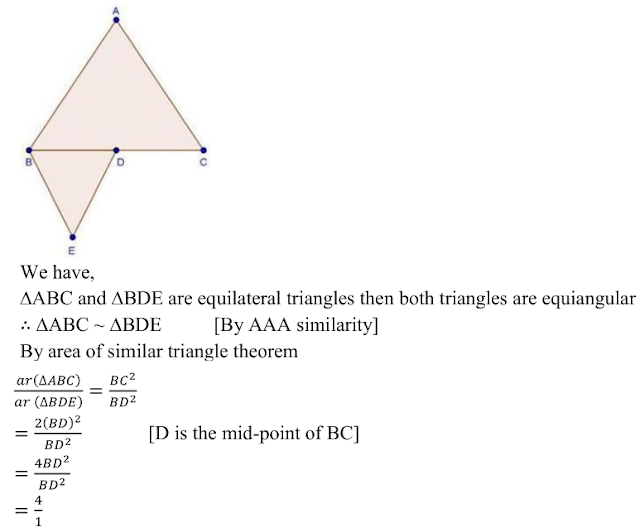18. Two isosceles triangles have equal vertical angles and their areas are in the ratio 36 : 25. Find the ratio of their corresponding heights.

Solution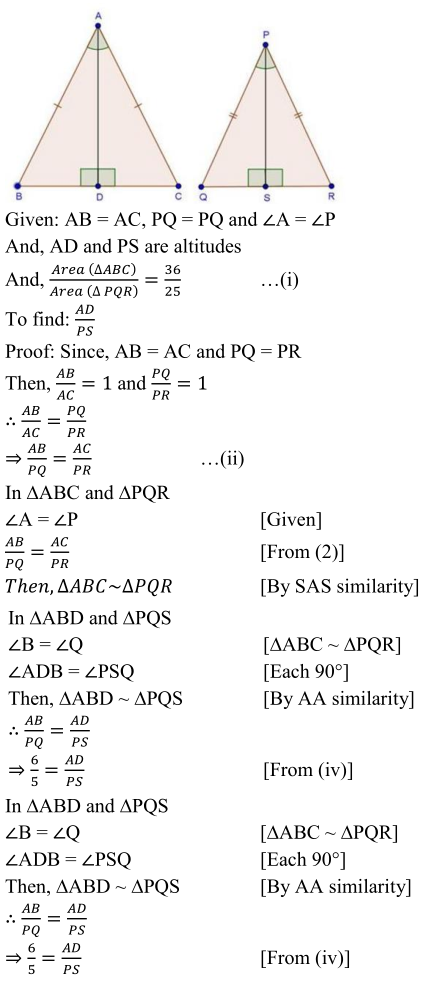19. In Fig., 4.180, ∆ABC and ∆DBC are on the same base BC. If AD and BC intersect at O, prove that Area(∆ABC)/Area(∆DBC) = AO/DO .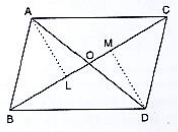Solution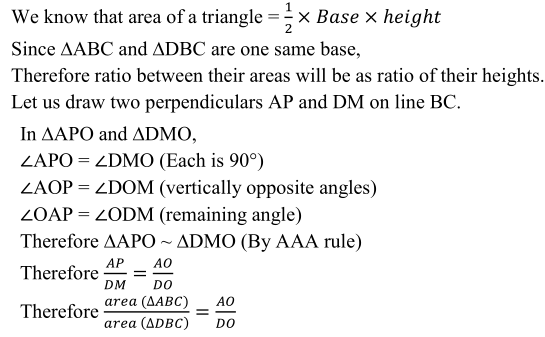20. ABCD is a trapezium in which AB || CD. The diagonals AC and BD intersect at O. Prove
that: (i) ∆AOB and ∆COD (ii) If OA = 6 cm, OC = 8 cm,
Find :
(a) area(∆AOB)area(∆COD)
(b) area(∆AOD)area(∆COD)

Solution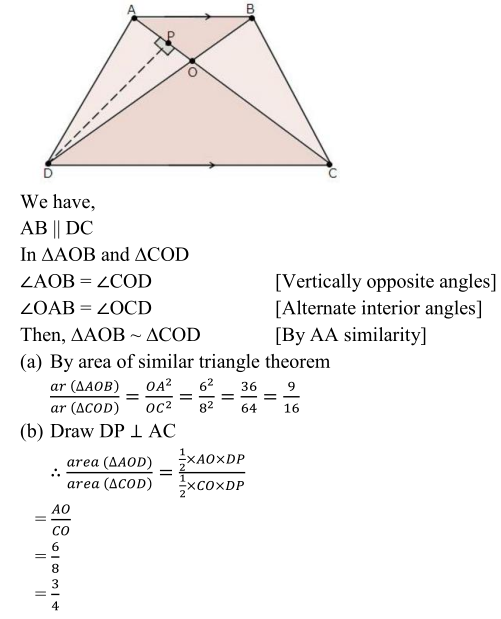21. In ABC, P divides the side AB such that AP : PB = 1 : 2. Q is a point in AC such that PQ || BC. Find the ratio of the areas of ∆APQ and trapezium BPQC.

Solution22. AD is an altitude of an equilateral triangle ABC. On AD as base, another equilateral triangle ADE is constructed. Prove that Area (∆ADE): Area (∆ABC) = 3: 4.

Solution